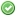rfc:instance_counter

This is an old revision of the document!

# PHP RFC: instance counter

## Introduction

Coming to Object-Orientated Programming, PHP offers a variety of possibilities to obtain information about classes and their instances (objects). In this regard, the classes/objects functions (http://www.php.net/manual/en/ref.classobj.php) as well as the reflection API (http://www.php.net/manual/en/book.reflection.php) do a great job. But still there is no function to obtain a particular information: the number of instances of a class.

One might say, simply implement a static counter in each class. But what about built-in classes like SPL? A wrapper would be required. Implementing it these ways, it not only sounds, but actually is cumbersome. The purpose of this RFC is to address this issue.

## Proposal

The proposal is to add a new functionality dealing with the number of instances of either a specific class name/object or set of class names/objects or all classes.

The function name should fit in with the current names of class/object functions. Therefore, the name get_objects_count() seems to be reasonable.

• If no argument is provided, the number of all objects in the object store as an associative array ('class name' ⇒ count) will be returned.
```print_r (get_objects_count());
// Array ()

\$foo = new stdClass;

print_r (get_objects_count());
// Array ( [stdClass] => 1 )

\$bar = new stdClass;

print_r (get_objects_count());
// Array ( [stdClass] => 2 )

\$bar = null;

print_r (get_objects_count());
// Array ( [stdClass] => 1 )

\$foo = null;

print_r (get_objects_count());
// Array ()```
• If a class name is provided, the number of objects of the specified class in the object store will be returned.
```print get_objects_count('stdClass');
// 0

\$foo = new stdClass;

print get_objects_count('stdClass');
// 1

\$bar = new stdClass;

print get_objects_count('stdClass');
// 2

\$bar = null;

print get_objects_count('stdClass');
// 1

\$foo = null;

print get_objects_count('stdClass');
// 0```
• If an object is provided, the number of objects of the specifiied objects class in the object store will be returned. The return value is always ≥ 1.
```\$foo = new stdClass;

print get_objects_count(\$foo);
// 1

\$bar = new stdClass;

print get_objects_count(\$bar);
// 2

\$bar = null;

print get_objects_count(\$foo);
// 1```
• If an an array is provided, it will be the treated as an inclusive indexed array of class names. An associative array ('class name' ⇒ count) will be returned.
```print_r (get_objects_count(array('stdClass')));
// Array ( [stdClass] => 0 )

\$foo = new stdClass;

print_r (get_objects_count(array('stdClass')));
// Array ( [stdClass] => 1 )

\$bar = new stdClass;

print_r (get_objects_count(array('stdClass')));
// Array ( [stdClass] => 2 )

\$bar = null;

print_r (get_objects_count(array('stdClass')));
// Array ( [stdClass] => 1 )

\$foo = null;

print_r (get_objects_count(array('stdClass')));
// Array ( [stdClass] => 0 )```

### Inheritance

On internals list there was the question, if only the “direct” instances of a class or also the instances of subclasses are counted? The answer is: only direct instances. See the following code:

```class A {}
class B extends A {}

print get_objects_count('A');
// 0

\$b = new B;

var_dump(\$b instanceof A);
// bool(true)

print get_objects_count('A');
// 0

print get_objects_count('B');
// 1```

## Use cases:

• Debugging
• Implementation of design patterns (f.ex. flyweight pattern)
• Extended control: limit the number of objects
• Teach people about assignment of objects
• to be continued…

## Function name

Since there were no objections against the proposed function name, its status changes to accepted.

```// Proposed + Accepted
get_objects_count()

// Alternatives
get_instances_counts()
get_instances_count()
get_instance_counts()
get_object_store_count()
get_class_counts() ```

No BC breaks.

## Proposed PHP Version(s)

next PHP 5.4.x or PHP 5.5.x

None.

None.

None.

None.

None.

## Patches and Tests

Implementation: https://gist.github.com/krakjoe/5275773

Tests need to be done.

- None.

## Vote

Should this RFC be implemented?
Real name Yes No
aharvey (aharvey)derick (derick)dm (dm)ircmaxell (ircmaxell)laruence (laruence)levim (levim)lstrojny (lstrojny)nikic (nikic)pajoye (pajoye)pierrick (pierrick)reeze (reeze)salathe (salathe)sebastian (sebastian)stas (stas)tyrael (tyrael)Count: 1 14

### Voting period

Start: 2013/04/30

End: 2013/05/07

### Page Tools﻿